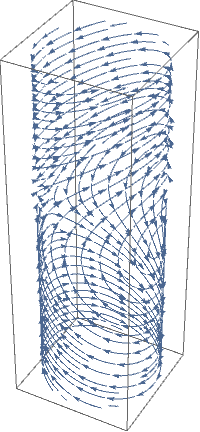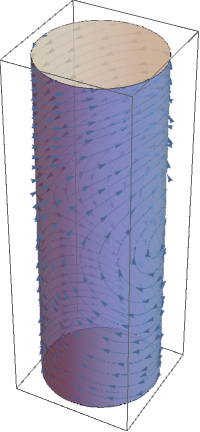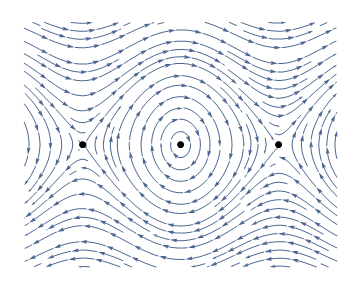# Preface

This section is about phase portrait for the pendulum equarion.

Introduction to Linear Algebra with Mathematica

# Phase Pendulum Portrait

Example: Consider the matrix You can draw phase portrait for the pendulum not on the plane $$\mathbb{R}^2 ,$$ but on the cylinder $$S^1 \times \mathbb{R} .$$ The pendulum of course has only two equilibria, one at (0,0) and another one at (π,0).
plot = StreamPlot[{y, -Sin[x]}, {x, -Pi, Pi}, {y, -3, 3}, Frame -> None, Epilog -> {PointSize -> Large, Point[{{0, 0}, {π, 0}, {-π, 0}}]}, StreamPoints -> Fine, AspectRatio -> 0.8]
First[Normal@plot] /. a_Arrow :> ( a /. {x_Real, y_Real} :> {Cos[x], Sin[x], y} ) // Graphics3DShow[ %, Graphics3D@{Opacity@.7, LightBlue, Cylinder[{{0, 0, -3}, {0, 0, 3}}]} ]img = Image@StreamPlot[{y, -Sin[x]}, {x, -5, 5}, {y, -3, 3}, Frame -> None, PlotRange -> {{-5, 5}, {-3, 3}}, Epilog -> {PointSize -> Large, Point[{{0, 0}, {Pi, 0}, {-Pi, 0}}]}, StreamPoints -> Fine, AspectRatio -> 0.8, PlotRangePadding -> 0, ImageMargins -> 0, ImageSize -> 800];

Graphics3D[{Texture[img], EdgeForm[], cyl[{{0, 0, 0}, {0, 0, 2 Pi}}, 1]}, Boxed -> False]
img = Rasterize[ StreamPlot[{y, -Sin[x]}, {x, -5, 5}, {y, -3, 3}, Frame -> None, PlotRange -> {{-5, 5}, {-3, 3}}, Epilog -> {PointSize -> Large, Point[{{0, 0}, {Pi, 0}, {-Pi, 0}}]}, StreamPoints -> Fine, AspectRatio -> 0.8, PlotRangePadding -> 0, ImageMargins -> 0, ImageSize -> 500], Background -> None, ImageResolution -> 300];

Graphics3D[{Texture[ImageData@img], EdgeForm[], Cylinder[{{0, 0, 0}, {0, 0, 2 Pi}}, 1]}, Boxed -> False, Lighting -> "Neutral"]

The vector field can also be interpreted as a velocity vector field. This means that a point x in the phase space moves along a trajectory so that its velocity vector at each instant equals the vector of the vector field attached to the location of x. Such a trajectory X(t), also called an orbit, trajectory, streamline, is simply the solution of an ordinary differential equation
$\dot{\bf x} = {\bf f}({\bf x}) ,$
where f is the vector field defined by $${\bf f} ({\bf x}) = (y, -\sin x ) .$$ It is however more convenient to represent the trajectories on a plane instead of on a cylinder. This can be done by expanding the cylindrical phase space by periodicity onto a phase plane. The following diagram is called a phase portrait.
StreamPlot[{y, -Sin[x]}, {x, -5, 5}, {y, -3, 3}, Frame -> None, StreamPoints -> Fine, AspectRatio -> 0.8, Epilog -> {PointSize -> Large, Point[{{0, 0}, {$Pi], 0}, {-\[Pi], 0}}]}]A phase point x is at equilibrium if the vector field cancels at this point, that is to say \[ \dot{\bf x} = {\bf f}({\bf x}) =0 \qquad \Longrightarrow \qquad \begin{cases} y&=0 , \\ \sin x &=0 . \end{cases}$
Solving the latter, we get equilibrium points:
$\begin{cases} y&=0 , \\ x &=n\pi , \quad \mbox{with n integer} . \end{cases}$